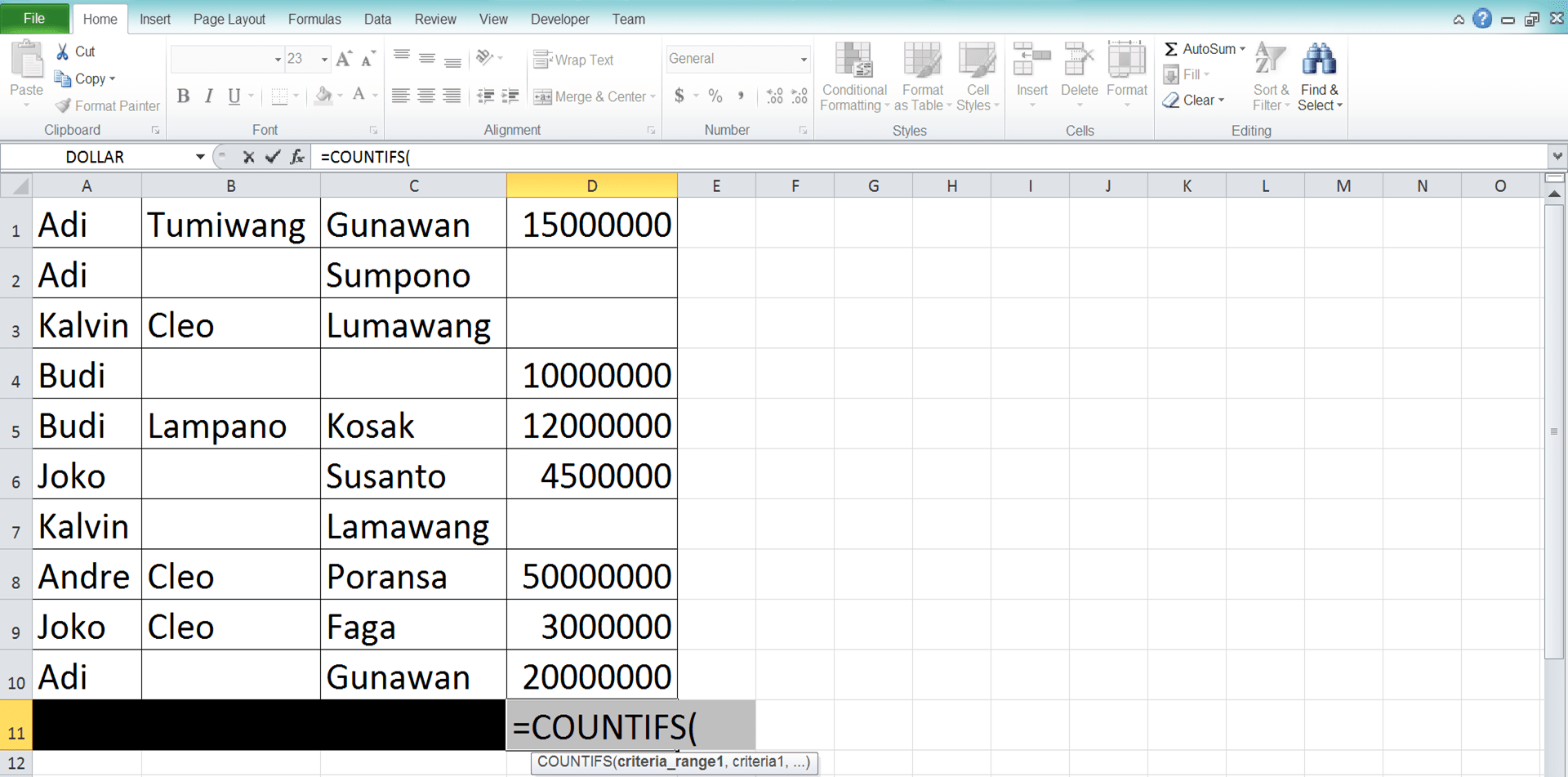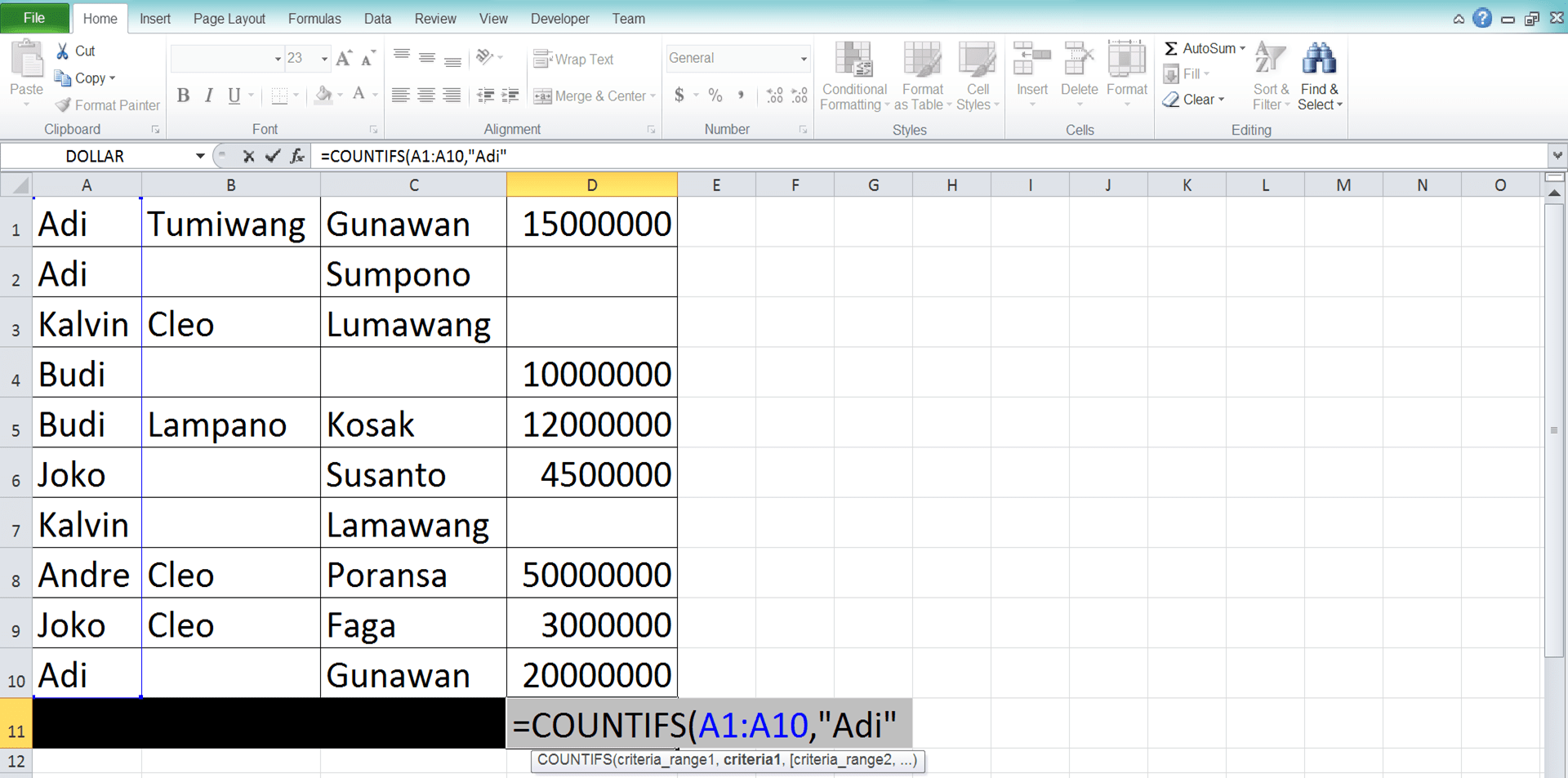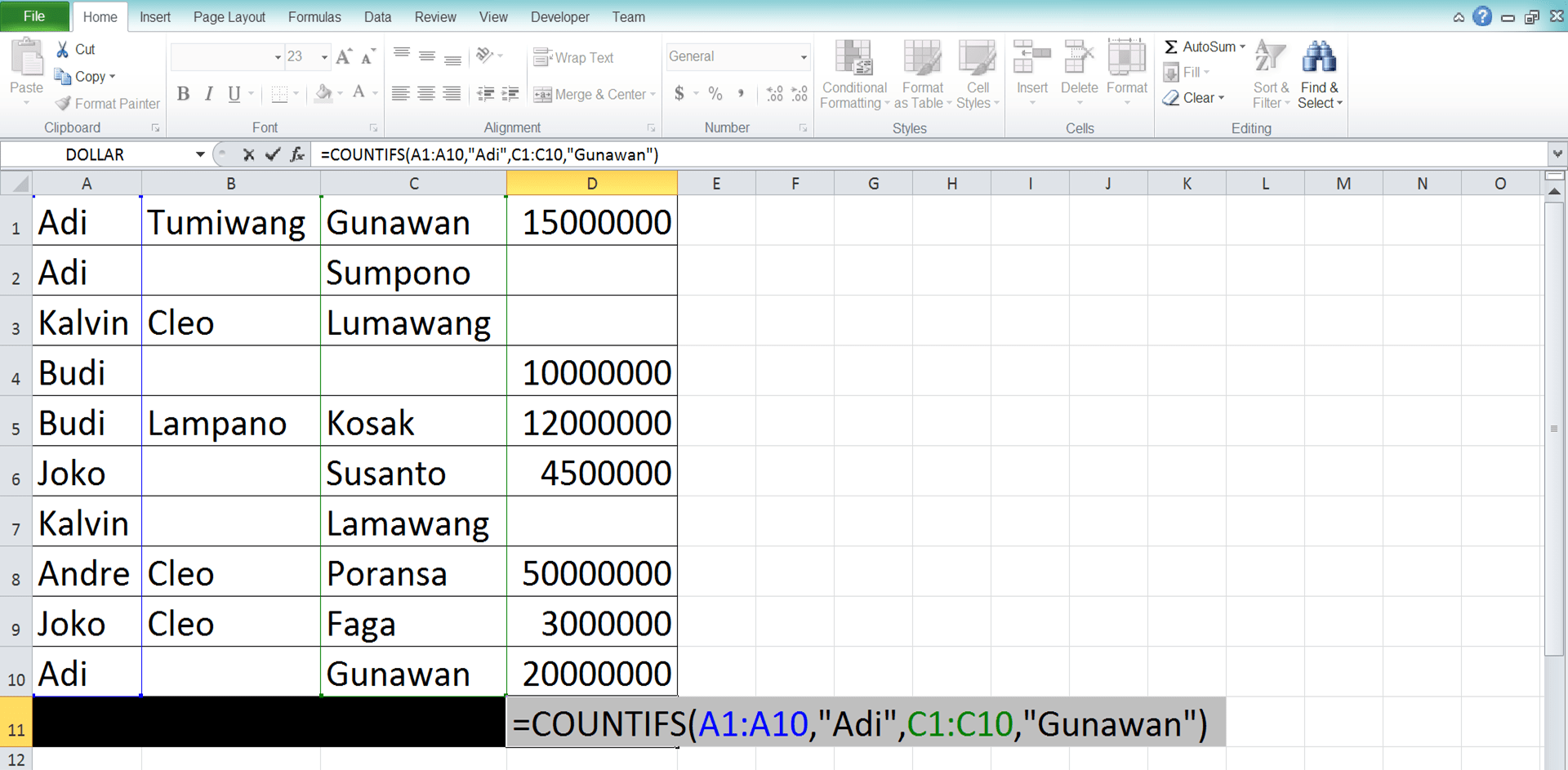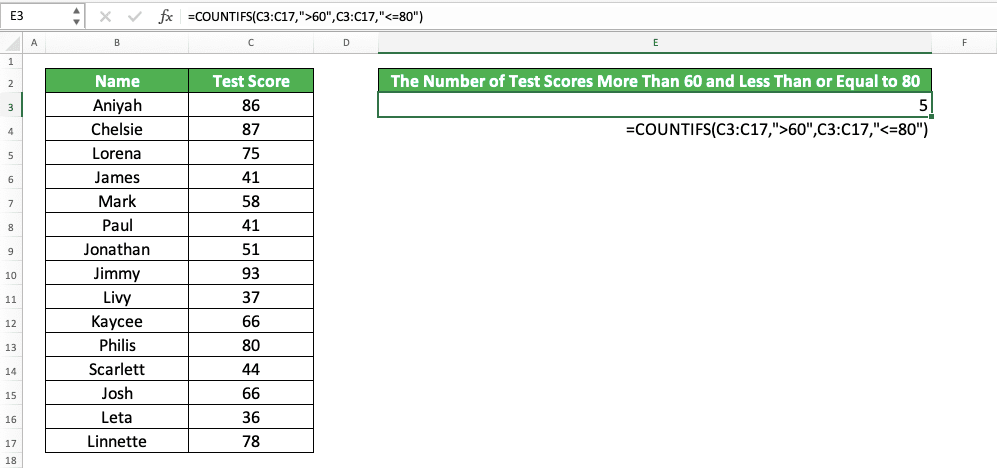How to Use COUNTIFS Excel Formula: Function, Examples, and Writing Steps - Compute Expert

# How to Use COUNTIFS Excel Formula: Function, Examples, and Writing Steps

Home >> Excel Tutorials from Compute Expert >> Excel Formulas List >> How to Use COUNTIFS Excel Formula: Function, Examples, and Writing Steps

In this tutorial, you will learn completely how to use the COUNTIFS excel formula.

COUNTIFS is a formula that can be very important in your data processing. Its ability to count the data you have based on your preferred criteria surely can help in various ways.

Want to know all about the formula usage in excel? Follow this tutorial until its last part!

Disclaimer: This post may contain affiliate links from which we earn commission from qualifying purchases/actions at no additional cost for you. Learn more

## COUNTIFS Excel Function

We can use COUNTIFS to count data in a cell range that meets our criteria.

## COUNTIFS Result

The result of COUNTIFS is a number that represents the amount of data that meet our criteria in a cell range.

## Excel Version from Which COUNTIFS can be Used

We can use the COUNTIFS formula in excel since excel 2007.

## The Way to Write It and Its Inputs

Generally, the writing form of COUNTIFS in excel can be illustrated as follows.

= COUNTIFS ( criteria_range1, criteria1, … )

A brief description of the inputs is as follows.
• criteria_range1 = the cell range which data you want to evaluate with your first criterion
• criteria1 = your first criterion
• = other cell ranges and criteria pairings

It is important to not forget that you should input parallel and same size cell ranges in COUNTIFS. This is so you don’t get an error from your COUNTIFS and you can easily check the COUNTIFS result if needed.

Usually, you input cell ranges in COUNTIFS in the form of parallel rows or columns.

## Example of Its Usage and Result

You can see the implementation and result example of excel COUNTIFS formula in the screenshot below.In the example, you can see directly the writing sample and result of the COUNTIFS. You must give the inputs mentioned in the previous tutorial section to the COUNTIFS. They are the cell ranges in which data will be evaluated with their pairing criteria and the criteria themselves.

Later, COUNTIFS will count the data entries which data meet the criteria in all of their cell ranges. We can see how this process runs and produces from the result in the example. There, only the respondents with the B, A, and C answers are counted by COUNTIFS (3).

For the cell range inputs, don’t forget that all of them must have the same size. Moreover, they should be in line with one another so it is easier to check the result logic if needed.

## Criteria Writing in COUNTIFS

For the COUNTIFS criteria inputs, there are many writing forms you can use, depending on the kinds of criteria you have.

You can see these criteria writings examples and their meaning below.

Text (not case-sensitive)
Criterion ExampleExplanation
"Jim"The same as “Jim”
“<>Jim”Not the same as “Jim”
“Jim*”With “Jim” prefix
“*jim”With “jim” suffix
“J*m”“J” prefix and “m” suffix
“Jim?”“Jim” prefix with any one character suffix
“?jim”Any one character prefix with “jim” suffix
“J?m”“J” prefix, any one character, and “m” suffix
“Jim~*”The same as “Jim*”
“Jim~?”The same as “Jim?”

A bit explanation of the symbols used in some of the text criterion:
• * = any character with any amount
• ? = any one character
• ~ = used when you want to add a * or ? character in the criterion

Number
Criterion ExampleExplanation
70Equal to 70
“>70”More than 70
“<70”Less than 70
“>=70”More than or equal to 70
“<=70”Less than or equal to 70

Date
Criterion ExampleExplanation
“>”&DATE(2019,12,3)Later than 3 December 2019

It isn’t recommended to input a date criterion with direct writing (e.g. “>3-12-2019”). The reason is that it can produce a wrong and unexpected result.

Cell coordinate
Criterion ExampleExplanation
“>”&B1More than the value in B1

Empty/non-empty cell
Criterion ExampleExplanation
“"Empty
“<>"Not empty

## Writing Steps

After learning the COUNTIFS implementation example and criteria writing, now let’s understand the COUNTIFS writing steps in excel. We will discuss each writing step together with its screenshot example to make you understand the steps much easier.

1. Type an equal sign ( = ) in the cell where you want to put the COUNTIFS result in2. Type COUNTIFS (can be with large and small letters) and an open bracket sign after =3. Input the cell range which data you want to evaluate with your first criterion. Then, type a comma sign ( , )5. Optional: If there are still other criteria you want to involve in the data counting process, then do this. Type a comma sign, then redo steps 3-4. Do this repeatedly until you have inputted all your data counting criteria6. Type a close bracket sign after you have inputted all the cell range and criterion pairings7. Press Enter
8. The process is done!## Most Possible Reasons Why Your COUNTIFS Produces an Error/Wrong Result

Got a wrong result from your COUNTIFS after you check it? Or even an error?

There can be many factors that make your COUNTIFS produce the kind of result you don’t want. However, these factors can be the most often reasons why that happens.
• You don’t write your criteria correctly. Take a look again at your COUNTIFS criteria inputs and the criteria writing examples we discuss previously. Have you got it all correct in your criteria writings?
• You wrongly pair your cell ranges and criteria. Remember that the criterion you input in COUNTIFS only evaluates data in the cell range you input before it. Wrong order of inputs can result in a skewed result from your COUNTIFS
• The cell ranges you input aren’t in the same size and/or aren’t parallel with each other

Check again your COUNTIFS writing and make sure you don’t do the mistakes above!

## COUNTIFS to Count Numbers in a Range

One of the common implementations for COUNTIFS in excel is to count numbers in a range.

If you, for example, need to count numbers between 5 and 25 in your cell range, you need to use COUNTIFS. That is because you cannot input a number range criterion in one writing in excel. You need to split the range into “more than (and equal to)” and “less than (and equal to)” criteria.

Here is the general writing form of COUNTIFS to count the numbers you have in a range.

= COUNTIFS ( number_range, “>lower_range_limit”, number_range, “<upper_range_limit” )

The number range input there is the cell range where the numbers you want to count based on your range are. You most often input the same cell range for the two instances of the number range in the COUNTIFS there (because you evaluate the same cell range to count the numbers in the lower and upper range limit criteria range).

You can change the “>” and “<“ there into “>=“ and “<=“ if you need to have an “equal to” criterion too for those limits.

To make the concept easier to understand, here is an example of its implementation in excel.In the example, we try to find the number of test scores that fall in the range of 60-80 (excluding 60). To get the answer for this, we use COUNTIFS.

In the COUNTIFS, we input the cell range of the test score column twice. Following each test score column cell range is the “more than” and “less than equal to” criteria.

We adjust those criteria to reflect the range we want. Thus, we write more than 60 (“>60”) and less than equal to 80 criteria there (“<=80”).

And so, that’s how we input the number range criterion in COUNTIFS. As you can see in the screenshot, we get the count result we need from our COUNTIFS writing!

## COUNTIF and COUNTIFS Difference

Before you learn COUNTIFS in this tutorial, you may have learned about COUNTIF. You can also use COUNTIF to count data in a cell range based on a criterion you have.

Thus, what is the difference between the two formulas, and which one should you use? Well, as you may have already known, COUNTIF can only count data based on one criterion. On the other hand, COUNTIFS can count data based on one or more criteria.

One or more criteria? Does it mean you can also use COUNTIFS to run a COUNTIF function?

The answer for that question is yes. If you only have one criterion for your data counting in excel, then you can use COUNTIF or COUNTIFS. However, if you have more than one criterion, then you need to use COUNTIFS.

It is a different case if you have excel 2003, of course. If you have that version of Excel, then you can only use COUNTIF since COUNTIFS is only available since excel 2007.

## Exercise

After you learn how to use the COUNTIFS excel formula, you can practice your understanding through this exercise!

### Questions

1. How many rows with the letter A in the first and second columns?
2. How many rows with the letter C in the second and third columns?
3. How many rows with the letter A in the first column, B in the second column, and C in the third column?

You can input up to 127 cell ranges and criteria pairings in COUNTIFS.

Related tutorials you should learn:

Get updated excel info from Compute Expert by registering your email. It's free!